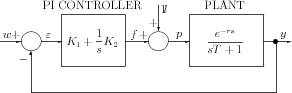]> 3.1.1 Example 1: Nuclear reactor temperature control I

#### 3.1.1 Example 1: Nuclear reactor temperature control I

Consider the nuclear reactor temperature control system depicted in Figure 3.1.Figure 3.1: The nuclear reactor temperature control system

The dynamics equations of the system described in  are

 $\left\{\begin{array}{ccc}\hfill Tẏ\left(t\right)+y\left(t\right)& \hfill =\hfill & p\left(t-r\right)\hfill \\ \hfill 1l\left(t\right)+f\left(t\right)& \hfill =\hfill & p\left(t\right)\hfill \\ \hfill {K}_{1}\varepsilon \left(t\right)+{K}_{2}{\int }_{0}^{t}\varepsilon \left(\tau \right)d\tau & \hfill =\hfill & f\left(t\right)\hfill \\ \hfill w-y\left(t\right)& \hfill =\hfill & \varepsilon \left(t\right)\hfill \end{array}\right\},\phantom{\rule{2em}{0ex}}t>0$ (3.1)

where ${K}_{1}$, ${K}_{2}$ are parameters, $1l$ denotes the Heaviside step function and $r$, $T$ are ﬁxed positive constants. If we assume that the system is asymptotically stable and until the moment of the appearance of a disturbance it remains in equilibrium, then for $t<0$

 $\varepsilon =0,\phantom{\rule{2em}{0ex}}f={K}_{2}{\int }_{0}^{\infty }\varepsilon \left(t\right)dt=w,\phantom{\rule{2em}{0ex}}p=w,\phantom{\rule{2em}{0ex}}y=w$ (3.2)

From (3.1) and (3.2) we get

$\stackrel{̈}{\varepsilon }\left(t\right)=-\frac{1}{T}\stackrel{̇}{\varepsilon }\left(t\right)-\frac{1}{T}\delta \left(t-r\right)-\frac{{K}_{1}}{T}\stackrel{̇}{\varepsilon }\left(t-r\right)-\frac{{K}_{2}}{T}\varepsilon \left(t-r\right)$

where $\delta$ denotes Dirac’s pseudofunction, together with the initial conditions

$\varepsilon \left(\theta \right)=0,\phantom{\rule{1em}{0ex}}\stackrel{̇}{\varepsilon }\left(\theta \right)=0\phantom{\rule{2em}{0ex}}\text{for}\phantom{\rule{2em}{0ex}}\theta \in \left[-r,0\right].$

Hence, introducing the state variables ${z}_{1}\left(t\right)=\varepsilon \left(t+r\right)$, ${z}_{2}\left(t\right)=\stackrel{̇}{\varepsilon }\left(t+r\right)$ and the notation

${z}_{2}^{0}=a\ne 0,\phantom{\rule{2em}{0ex}}a=-\frac{1}{T},\phantom{\rule{2em}{0ex}}b=-\frac{{K}_{1}}{T}=-5{K}_{1},\phantom{\rule{2em}{0ex}}d=-\frac{{K}_{2}}{T}=-5{K}_{2}$

we obtain the ﬁnal version of the dynamics equations

 $\left\{\begin{array}{cccc}\hfill {ż}_{1}\left(t\right)& \hfill =\hfill & {z}_{2}\left(t\right)\hfill & \hfill \\ \hfill {ż}_{2}\left(t\right)& \hfill =\hfill & a{z}_{2}\left(t\right)+b{z}_{2}\left(t-r\right)+d{z}_{1}\left(t-r\right)\hfill & \hfill \\ \hfill {z}_{1}\left(\theta \right)& \hfill =\hfill & 0,\hfill & -r\le \theta \le 0\hfill \\ \hfill {z}_{2}\left(\theta \right)& \hfill =\hfill & 0,\hfill & -r\le \theta <0\hfill \\ \hfill {z}_{2}\left(0\right)& \hfill =\hfill & {z}_{2}^{0}\hfill \end{array}\right\}$ (3.3)

The problem is to determine a pair $\left(b,d\right)$ minimizing the integral performance index

 $J={\int }_{0}^{\infty }{\varepsilon }^{2}\left(t\right)dt={\int }_{0}^{\infty }{\varepsilon }^{2}\left(t+r\right)dt={\int }_{0}^{\infty }{z}_{1}^{2}\left(t\right)dt\phantom{\rule{2em}{0ex}}\left(\varepsilon \equiv 0\phantom{\rule{1em}{0ex}}\text{on}\phantom{\rule{1em}{0ex}}\left[-r,0\right]\right)$ (3.4)

The system (3.3) is a special case of the neutral system

 (3.5)

where ${A}_{1}$, ${A}_{2}$, ${A}_{0}\in L\left({\mathbb{ℝ}}^{n}\right)$, $r>0$, ${v}_{0}\in {\mathbb{ℝ}}^{n}$, $\phi$ is a function deﬁned on $\left(-r,0\right)$ with values in ${\mathbb{ℝ}}^{n}$. This can be seen by taking

$\begin{array}{c}n=2,\phantom{\rule{2em}{0ex}}{A}_{1}=\left[\begin{array}{cc}\hfill 0& \hfill 1\\ \hfill 0& \hfill a\end{array}\right],\phantom{\rule{2em}{0ex}}{A}_{2}=\left[\begin{array}{cc}\hfill 0& \hfill 0\\ \hfill d& \hfill b\end{array}\right],\phantom{\rule{2em}{0ex}}{A}_{0}=0\in L\left({\mathbb{ℝ}}^{2}\right),\phantom{\rule{1em}{0ex}}\left(v=z\right),\hfill \\ {v}_{0}=\left[\begin{array}{c}\hfill 0\hfill \\ \hfill {z}_{2}^{0}\hfill \end{array}\right],\phantom{\rule{2em}{0ex}}\phi \equiv 0.\hfill \end{array}$